# Jernej Barbic's Research Code

Vega FEM 4.0 has been released. Vega is a computationally efficient and stable C/C++ library to timestep nonlinear three-dimensional deformable models. It is designed to model large deformations, including geometric and material nonlinearities, and can also efficiently simulate linear systems.

Much of the code below is now available in Vega FEM.

All code is C/C++, and released under the BSD license.

All code written by Jernej Barbic. Joint research with Prof Doug L. James, at the Carnegie Mellon University Graphics Lab and Prof Jovan Popovic, at the MIT Computer Graphics Group.

If you publish a research paper using this code, we will appreciate if you cite our implementation (for example, like this), or you can cite one of our relevant papers. This code is research code. If you find bugs, please let us know.

This code is cross-platform. It compiles under Mac OS X, Linux, and Windows. Please do not repost this code on other websites, in any format.

This material is based upon work supported by the National Science Foundation under Grant No. CAREER-53-4509-6600. Any opinions, findings and conclusions or recomendations expressed in this material are those of the author(s) and do not necessarily reflect the views of the National Science Foundation (NSF). This work was also supported by the James H. Zumberge Research and Innovation Fund at USC.

Feedback form

## StVK: FEM Saint Venant-Kirchhoff deformable object library (multi-threaded)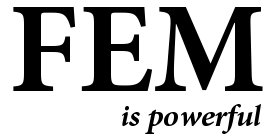This class can compute FEM internal forces, tangent stiffness matrices, and the Hessians of the internal forces, for 3D volumetric meshes (for full models, without any reduction). Two mesh element types are supported: linear tetrahedra and cubes (i.e., the two types of meshes supported by our "volumetricMesh" class). Large deformations are supported ("geometric nonlinearities"). The strain-stress law is linear (the Saint Venant-Kirchhoff model). Implementation is multi-threaded and achieves near-linear scalability (tested on an Intel Xeon dual-processor, with each processor a quad-core (8 cores total)).

This library also supports model reduction. It can precompute the cubic polynomials of a reduced StVK deformable model. It can also evaluate the reduced internal forces, stiffness matrices, and Hessians very efficiently.

Dependencies: volumetricMesh, sparseMatrix.
This library is a part of Vega. Look for it in the "libraries/stvk" folder in Vega.

## Modal Matrix: A class for modal matrix operations (assembly u=Uq, projection q = U^T u, optionally with SSE instructions)

Given a modal matrix U, this class can perform multiplications u=Uq, and q=U^Tu. These are standard operations frequently encountered in modal simulations.

Dependencies: none
This library is a part of Vega. Look for it in the "libraries/modalMatrix" folder in Vega.

## Mass Spring System: A general 3D mass-spring system (multi-threaded)

This class allows you to compute mass spring system internal forces, tangent stiffness matrices, and Hessians of internal forces. It can also compute damping. Arbitrary 3D mass-spring networks are supported. You specify the vertices and connectivity, and this class provides forces, stiffness matrices, and Hessians. It is also possible to build a mass-spring network directly from a tetrahedral mesh (vertices become masses, and edges become springs).

Dependencies: volumetricMesh, sparseMatrix, configFile.
This library is a part of Vega. Look for it in the "libraries/massSpringSystem" folder in Vega. Acknowledging

## Implicit Newmark and Central Differences IntegratorsThis code can numerically timestep large deformation nonlinear elasticity. More specifically, this code can numerically timestep a system of ODEs of the form:

M * q'' + (alpha * M + beta * K(q)) q' + R(q) = fext(t),

where q is an unknown vector function (such as the deformations), fext(t) are arbitrary user-provided external forces, R(q) is an arbitrary user-provided vector function, and K(q) = d R / d q is its user-provided gradient. Parameters alpha and beta are Rayleigh damping parameters. Such ODEs are obtained when simulating nonlinear FEM elasticity of large-deformation deformable models: R(q) are the internal elastic forces, and K(q) is the tangent stiffness matrix.

Two integrators are provided: implicit Newmark (implicit), and central differences (explicit). Implicit Newmark is stable even with large timesteps, but requires a system solve at every timestep, and (especially with large timesteps) introduces some "numerical viscosity" into the solution. Central differences require very small timesteps to be stable. The library supports both large sparse systems (arising with geometrically detailed deformable meshes), and small dense systems (arising with model reduction of large sparse systems). The code works in tandem with the StVK library: appropriate R(q) and K(q) functions are already provided both for large sparse elasticity and dense reduced systems.

The code uses either SPOOLES, PARDISO, or our own Jacobi-preconitioned conjugate gradient solver (see the "sparseMatrix" package) to solve the large sparse linear systems. For dense systems, it uses LAPACK.

Also provided are two driver examples. The first is a complete FEM StVK large sparse nonlinear elasticity simulator (does not use any reduction). The second example is a real-time deformable model simulator, designed based on model reduction.

With reduction, we used dense ODEs up to the size of about 60. Note that the computation slows down significantly with the size of the reduced basis; this limitation was addressed in the following paper: Steven An, Theodore Kim, Doug L. James: Optimizing Cubature for Efficient Integration of Subspace Deformations, ACM Transactions on Graphics (SIGGRAPH ASIA 2008), 27(5), December 2008.

Dependencies: BLAS, LAPACK, StVK.
Note: This library is now a part of Vega. Look for it in the "libraries/integrator" folder in Vega.

## Large Modal Deformation Factory: Model reduction of StVK FEM deformable models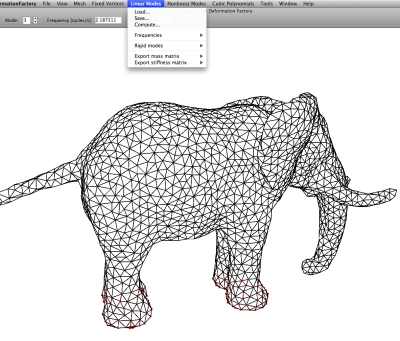Given a simulation mesh (tetrahedral or voxel), and a set of constrained vertices, this Windows application (complete, standalone, with an installer) can generate a fast reduced deformable model, as described in the following SIGGRAPH paper:

Jernej Barbic, Doug L. James: Real-Time Subspace Integration
for St.Venant-Kirchhoff Deformable Models, ACM Transactions on
Graphics (SIGGRAPH 2005), Los Angeles, CA, August 2005

The application starts either with a triangle mesh or a volumetric mesh, and generates all the necessary data for a subsequent real-time simulation. It can also launch such a simulation, using a provided real-time executable "stvk.exe". Or, you can run a real-time simulation from your own code using our C++ classes provided on this page ("newmark" and "StVK" libraries). The application supports tetrahedral and voxel 3D volumetric meshes. The application also contains several generally useful sub-components:
• compute linear modes (via ARPACK) of arbitrary volumetric (tet or voxel) meshes, under arbitrary boundary conditions (including free boundary conditions),
• compute modal derivatives,
• compute volumetric mesh mass and stiffness matrix,
• create cube volumetric meshes for arbitrary input triangle geometry, as described in this SIGGRAPH 2004 Sketch (loadable by our "VolumetricMesh" class).

The application outputs several files, which are compatible with the rest of the code available on this webpage. I.e., it produces the cubic polynomial of reduced internal forces that you can then load using the "StVKReducedInternalForces" and "ImplicitNewmark" classes (see the "StVK" and "newmark" libraries) and timestep in your own code. All the modal matrices generated (linear modes, modal derivatives, or simulation basis matrix) can be loaded using the "Matrix" class. Instructions are zipped with the executable.

If you want to use tetrahedral meshes, you can generate them using the TetGen mesh generation package.

Note: This executable is version 2.0. A better, more stable version (3.2) is available in Vega FEM 2.0. Full source code is available in Vega. Look for it in the "utilities/largeModalDeformationFactory" folder in Vega.
Note: This application comes with several example meshes. Look for them in the "example" subfolder of your install location. If you can't find them, they are here.

## iOS demo utility to play reduced deformable models. Written for iPhone 4S, in 2012.

This is a demo utility whereby the user can apply forces to the reduced deformable model by swiping their fingers across iPhone's screen.

Dependencies: none
ios-reducedStVK-v1.1.zip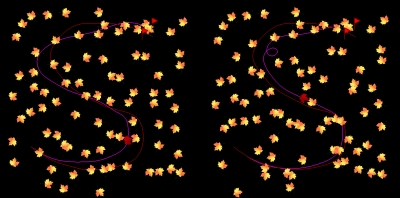The "LQR" class implements a linear-quadratic regulator (LQR) for the following linear time-varying dynamical system:

\dot{x} = F(t) * x + G(t) * u  ,


where x is the state, u is the control, and F and G are (potentially time-varying) matrices. F and G can be arbitrary (they are provided by you). The state and the control can have arbitrary dimensions (not necessarily equal). The implementation proceeds by solving a Riccati differential equation, backwards in time. In order to do so, it needs to compute exponentials of dense matrices. We compute these exponentials using the Expokit package.

Dependencies: matrix, expokit.
lqr-v1.0.zip

## Volumetric Mesh: tetrahedral and cube volumetric 3D meshes

 The classes in this library provide storage for a general volumetric 3D mesh. Two mesh types are supported: tetrahedral and voxel. The classes store the mesh geometric information (elements and vertices), and also the material parameters of each individual mesh element (Young's modulus, Poisson ratio, mass density). This is done by organizing elements with the same material parameters into a "solid section". The class supports several geometric queries and also interpolation to an embedded triangle mesh ("Free-Form Deformation"). It also supports exporting the mesh to an .ele or .node format (the format used, e.g., by the TetGen mesh generation package). The class can also compute the mass matrix of the given volumetric mesh. To generate a cube volumetricMesh from an input triangle mesh (optionally flood-filling interior chambers and/or including the interpolation weights), you can use the Large Modal Deformation Factory application. Dependencies: minivector, sparseMatrix (for mass matrix generation). Note: This library is now a part of Vega. Look for it in the "libraries/volumetricMesh" folder in Vega.## Cross-platform C code execution timer (performance counter)

A C++ counter to measure code execution time. The timer is accurate up to a few microseconds. You can time arbitrary segments of your code, by placing StartCounter() and StopCounter() before and after your code block. Same interface under Windows, Linux and Mac OS X. Under Windows, the timer uses "QueryPerformanceCounter", and under Linux/Mac OS X, it uses "gettimeofday".

Dependencies: none.
This library is now a part of Vega. Look for it in the "libraries/performanceCounter" folder in Vega. Acknowledging

## Matrix (dense)

The "Matrix" class implements a matrix: a 2D array of real values, together with the commonly defined algebraic operations. The matrix can be rectangular (need not be square). The matrix is stored in the column-major order (same as in LAPACK). Storage is dense (see the Sparse Matrix library for sparse matrices). The class suports common algebraic operations (addition, multiplication, transposition, etc.), including operator overloading, so you can write expressions like: A += 0.25 * (B + A) * C; . It is possible to load/save the matrix from/to a file, using a special well-documented binary format (see matrixIO.h). The class can also perform more complex matrix operations such as computing the SVD decomposition, solving linear systems (via LU decomposition), finding eigenvalues and eigenvectors, solving least square systems, and computing matrix inverses, pseudoinverses and exponentials. The code uses BLAS routines to perform matrix addition and multiplication, and LAPACK for SVD, LU, eigenanalysis, least square systems, inverses and pseudoinverses. Expokit is used for matrix exponentiation. The classes are templated for both float and double datatypes. Also included are routines to perform Principal Component Analysis (PCA) on the columns of the matrix.

Dependencies: BLAS, LAPACK, for matrix exponentiation also Expokit
This library is now a part of Vega. Look for it in the "libraries/matrix" folder in Vega. Acknowledging

## Sparse Matrix

 This class implements sparse matrices with the common algebraic operations such as incremental construction, addition, mtx-vec multiplication, load/save to disk, row-column deletion, etc. Suitable for large sparse matrices. Storage is per-row: for every matrix row, the class stores the integer indices of non-zero columns in that row, together with the corresponding matrix values. Also included is a Conjugate Gradient linear system solver (for positive-definite large sparse symmetric matrices), with (optional) Jacobi preconditioning. The CG Solver was implemented by following Jonathan Shewchuk's An Introduction to the Conjugate Gradient Method Without the Agonizing Pain. Also included is a Gauss-Seidel linear system solver. Dependencies: none. This library is a part of Vega. Look for it in the "libraries/sparseMatrix" and "libraries/sparseSolver" folder in Vega. Acknowledging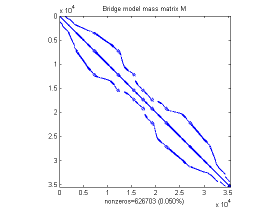## Minivector

A simple class for vector algebra on 2D vectors, 3D vectors (normalization, dot product, cross product, ...), and matrix algebra on 3x3 matrices (summation, multiplication, eigenvalue and eigenvector computation, ...).

Dependencies: none.
This library is now a part of Vega. Look for it in the "libraries/minivector" folder in Vega. Acknowledging

## Polar decomposition of a 3x3 matrix (and derivatives of polar decomposition matrices)

This class can compute the polar decomposition of a general 3x3 matrix. The polar decomposition code has been adapted from the (freely available) polar decomposition implementation provided as a companion to the book "Graphics Gems IV".

New (May 2011): The class can also compute the first and second derivatives of the matrices in polar decomposition, as explained in this publication.

Dependencies: minivector.
This library is now a part of Vega. Look for it in the "libraries/polarDecomposition" folder in Vega. Citation

## Dynamics of a (single) rigid body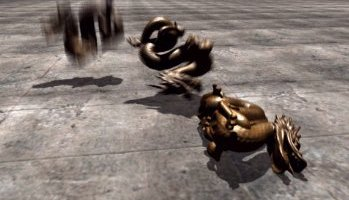These two classes ("RigidBody" and "RigidBody_GeneralTensor") implement 6-DOF rigid dynamics of a single rigid body, as explained in:

David Baraff:
"An Introduction to Physically Based Modeling:
Rigid Body Simulation I: Unconstrained Rigid Body Dynamics"
(SIGGRAPH 97 Course Notes)

These two classes allow you to simulate the motion of a single rigid body, under any specified (potentially time-varying) external forces and torques. Arbitrary tensors of inertia are supported. For rigid objects where the inertia tensor in the world coordinate system is diagonal, use "RigidBody". For the general case (non-diagonal inertia tensor in the world coordinate system), use "RigidBody_GeneralTensor". The solution is computed by numerically timestepping the ordinary differential equations of rigid body motion, derived from the Newton's 2nd law, and conservation of linear momentum and angular momentum. For example, ballistic motion can be simulated if gravity is used as the external force. Objects bouncing off the ground/impacting other objects can be simulated if you combine "RigidBody" (or "RigidBody_GeneralTensor") with a collision detection algorithm that provides the contact external forces.

Currently, the code supports explicit Euler integration and symplectic Euler.

Dependencies: quaternion.
This library is now a part of Vega. Look for it in the "libraries/rigidBodyDynamics" folder in Vega. Acknowledging

## Quaternions

This C++ class implements quaternions and the common algebraic operations on quaternions (including operator overloading). The class is templated: you can use either float or double precision. Supports using quaternions to represent/manipulate rotations. The Matrix2Quaternion routine is borrowed from David Baraff's course notes (with permission).

Dependencies: none.
This library is now a part of Vega. Look for it in the "libraries/quaternion" folder in Vega.

## A C++ class to (very quickly and exactly) timestep the following 1D ODE: M * q''(t) + C * q'(t) + K * q(t) = f(t)This class integrates a single harmonic oscillator, that is, this code can timestep the following one-dimensional Ordinary Differential Equation:

   M * q''(t) + C * q'(t) + K * q(t) = f(t),
where M,C,K are scalar constants, and
f=f(t) is a given (discretely sampled) function of time, and
q=q(t) is the unknown (i.e., what we are solving for).

The system must be underdamped (as is the case in, e.g., sound simulations):
C < 2 * sqrt(M*K).

The integration uses an IIR filter and is as such EXACT up to floating point arithmetic (i.e., this is better than integrating the ODE with a numerical integrator such as Euler/Runge-Kutta/Central Differences,etc.). The timestep computation is very simple and runs at very fast rates (easily at 44.1 kHz). This code can be used (and has been used) to integrate the modal oscillators for simulating sound, for example, in the following paper:
   Doug L. James, Jernej Barbic, Dinesh K. Pai:
Precomputed Acoustic Transfer: Output-sensitive, accurate sound generation
for geometrically complex vibration sources,
ACM Transactions on Graphics 25(3) (SIGGRAPH 2006),
p. 987-995, Boston, MA, August 2006.


Dependencies: none
harmonicOscillator_IIR-v1.0.zip

## A class to parse custom-defined text configuration files

This C++ class makes it possible to create your own text configuration file syntax, and then load the values from a particular configuration file (following your syntax) into variables of your C++ program. Supports int, float, double, bool, and char* datatypes.

This class works with text configuration files like these. The syntax of the file is defined using a C++ program in the following way. This example C++ program first defines the configuration file syntax. Then, it opens a particular configuration file and loads the values to the memory locations specified when the syntax was defined. The program output will be as follows.

For example, this can serve as an improved command-line option tool: you can move your command line options to a text file so that you don't have to retype them each time (useful if there are many). Or, it can serve as an interface between two programs. Write the values out to a file with one program and load them into another.

Dependencies: none.
This library is now a part of Vega. Look for it in the "libraries/configFile" folder in Vega. Acknowledging

## OpenGL lighting from a text configuration file

A class to read OpenGL lighting parameters from a text configuration file. Usage is simple: use the provided constructor to read the configuration file during initialization, then call "LightScene" inside your OpenGL display routine (after setting up the modelview and projection matrices). Now, you no longer have to recompile your code each time you wish to change some lighting parameter!

Here is an example configuration file.

Dependencies: configFile.
This library is now a part of Vega. Look for it in the "libraries/lighting" folder in Vega. Acknowledging

## OpenGL "spherical" camera

A class to position an OpenGL camera in 3D, using spherical coordinates. The camera points to a specified focus position.

Dependencies: none.
This library is now a part of Vega. Look for it in the "libraries/camera" folder in Vega. Acknowledging